mraa  2.0.0 Low Level Skeleton Library for Communication on GNU/Linux platforms
Pwm Class Reference

## Detailed Description

This file defines the PWM interface for libmraa

mraa::Pwm pwm(PWM_PORT);
std::cout << "Cycling PWM on IO3 (pwm3)" << std::endl;
pwm.enable(true);
while (flag) {
value = value + 0.01f;
pwm.write(value);
usleep(50000);
if (value >= 1.0f) {
value = 0.0f;
}
}

## Public Member Functions

Pwm (int pin, bool owner=true, int chipid=-1)

Pwm (void *pwm_context)

~Pwm ()

Result write (float percentage)

Result period (float period)

Result period_ms (int ms)

Result period_us (int us)

Result pulsewidth (float seconds)

Result pulsewidth_ms (int ms)

Result pulsewidth_us (int us)

Result enable (bool enable)

int max_period ()

int min_period ()

## Constructor & Destructor Documentation

 Pwm ( int pin, bool owner = `true`, int chipid = `-1` )
inline

instanciates a PWM object on a pin

Parameters
 pin the pin number used on your board owner if you are the owner of the pin the destructor will unexport the pin from sysfs, default behaviour is you are the owner if the pinmapper exported it chipid the pwmchip to use, use only in raw mode

Here is the call graph for this function: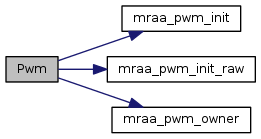Pwm ( void * pwm_context )
inline

Pwm constructor, takes a pointer to the PWM context and initialises the class

Parameters
 pwm_context void * to a PWM context
 ~Pwm ( )
inline

Pwm destructor

Here is the call graph for this function: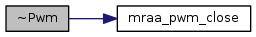## Member Function Documentation

 Result write ( float percentage )
inline

Set the output duty-cycle percentage, as a float

Parameters
 percentage A floating-point value representing percentage of output. The value should lie between 0.0f (representing 0%) and 1.0f Values above or below this range will be set at either 0.0f or 1.0f
Returns
Result of operation

Here is the call graph for this function: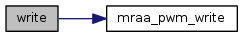float read ( )
inline

Read the output duty-cycle percentage, as a float

Returns
A floating-point value representing percentage of output. The value should lie between 0.0f (representing 0%) and 1.0f Values above or below this range will be set at either 0.0f or 1.0f

Here is the call graph for this function:Result period ( float period )
inline

Set the PWM period as seconds represented in a float

Parameters
 period Period represented as a float in seconds
Returns
Result of operation

Here is the call graph for this function:Result period_ms ( int ms )
inline

Set period, milliseconds

Parameters
 ms milliseconds for period
Returns
Result of operation

Here is the call graph for this function:Result period_us ( int us )
inline

Set period, microseconds

Parameters
 us microseconds as period
Returns
Result of operation

Here is the call graph for this function:Result pulsewidth ( float seconds )
inline

Set pulsewidth, as represented by seconds in a float

Parameters
 seconds The duration of a pulse
Returns
Result of operation

Here is the call graph for this function: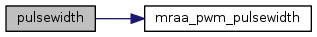Result pulsewidth_ms ( int ms )
inline

Set pulsewidth, milliseconds

Parameters
 ms milliseconds for pulsewidth
Returns
Result of operation

Here is the call graph for this function: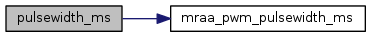Result pulsewidth_us ( int us )
inline

The pulsewidth, microseconds

Parameters
 us microseconds for pulsewidth
Returns
Result of operation

Here is the call graph for this function:Result enable ( bool enable )
inline

Set the enable status of the PWM pin. None zero will assume on with output being driven and 0 will disable the output

Parameters
 enable enable status of pin
Returns
Result of operation

Here is the call graph for this function:int max_period ( )
inline

Get the maximum PWM period in us

Returns
max PWM period in us

Here is the call graph for this function: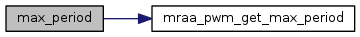int min_period ( )
inline

Get the minimum PWM period in us

Returns
min PWM period in us

Here is the call graph for this function: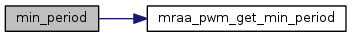The documentation for this class was generated from the following file: